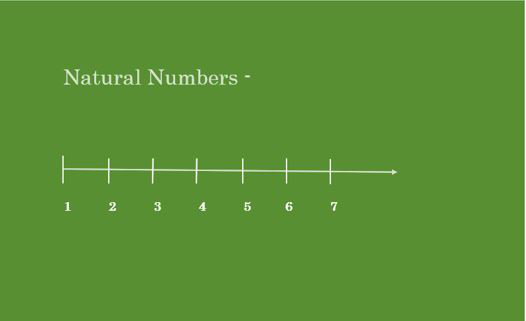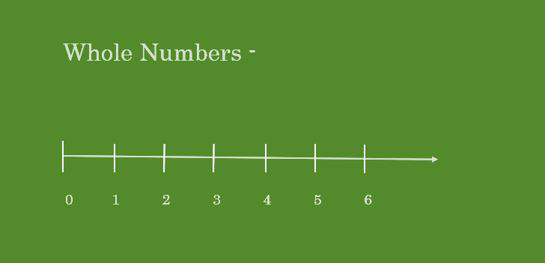Is 13 a whole number?

• Last Updated : 21 Sep, 2021

The method to represent and work with numbers is known as the number system. A number system is a system of writing to represent numbers. It is the mathematical notation used to represent numbers of a given set by using digits or other symbols. It has arithmetic operations to perform division, multiplication, addition, and subtraction between numbers. Some important number systems are as follows:

• Decimal Number System
• Binary Number System
• Octal Number System

Numbers and Digits

Numbers are the counts or measurements used in mathematics, numerals are used to define numbers. A numeral can be defined as a symbol used for counting, for instance, there are 55 books in the library, where 56 is the numeral which is a combination of digits 5 and 6. A digit is a single numeral, the combination of digits form numerals. In the decimal number system, there are 10 digits, they are 0, 1, 2, 3, 4, 5, 6, 7, 8, 9.

What are Naturals Numbers?

In the Number system, Natural numbers are the numbers that start from 1 and count up to Infinity. For example – (1, 2, 3, 4, 5, 6, 7, 8, 9, 10, 11,12, 13, 14, 15, 16, 17, 18, 19, 20, 21..…….. ∞) are Natural Numbers.What are whole numbers?

The Whole numbers are the natural or counting numbers including 0. Whole numbers are the numbers that start from 0 and count up to (∞) infinity Whole numbers are the subsets of natural numbers.

For example – (0, 1, 2, 3, 4, 5, 6, 7, 8, 9, 10, 11,12, 13, 14, 15……….. ∞) are Whole Numbers.

The first 50 whole numbers are: 0, 1, 2, 3, 4, 5, 6, 7, 8, 9, 10, 11, 12, 13, 14, 15, 16, 17, 18, 19, 20, 21, 22, 23, 24, 25,26, 27, 28, 29, 30, 31, 32, 33, 34, 35, 36, 37, 38, 39, 40, 41, 42, 43, 44, 45, 46, 47, 48, 49, 50.Is 13 a whole number?

Whole numbers are the set of natural numbers including 0 (zero) and all positive numbers whereas, excluding integers, decimals, and fractions. Example – 0, 1, 2, 3, 4, 5, 6….. etc.

Therefore 13 is a whole number because 13 is a part of all the natural numbers in the number system.

Similar Questions

Question 1. What is the smallest whole number?

Whole Numbers are {0, 1, 2, 3, 4, 5, 6, 7……, ∞}. Whole numbers are the numbers that are starting from 0 and counts to infinity. Therefore 0 is the smallest whole number.

Question 2. Check if 0.45 is a whole number?

0.45 is not a whole number because 0.45 is a decimal value and whole numbers are the set of real positive number excluding fraction, decimal, negative numbers i.e. 0.45 is not a whole number.

Question 3. Write all the whole numbers up to 50?

Whole numbers up to 50 are – 0, 1, 2, 3, 4, 5, 6, 7, 8, 9, 10, 11, 12, 13, 14, 15, 16, 17, 18, 19, 20, 21, 22, 23, 24, 25, 21, 22, 23, 24, 25,26, 27, 28, 29, 30, 31, 32, 33, 34, 35, 36, 37, 38, 39, 40, 41, 42, 43, 44, 45, 46, 47, 48, 49, 50.

Question 4. Is 7/2 a whole number?